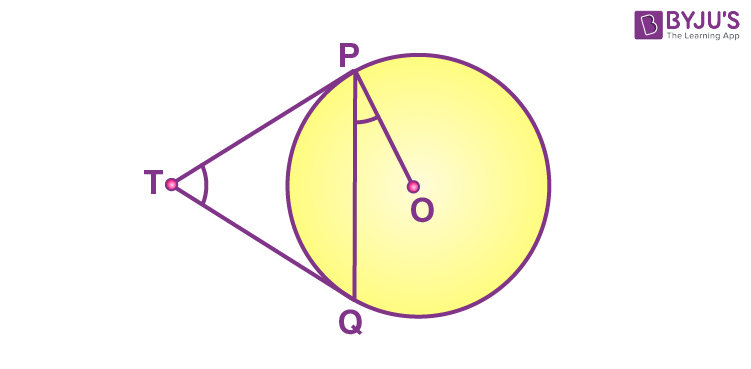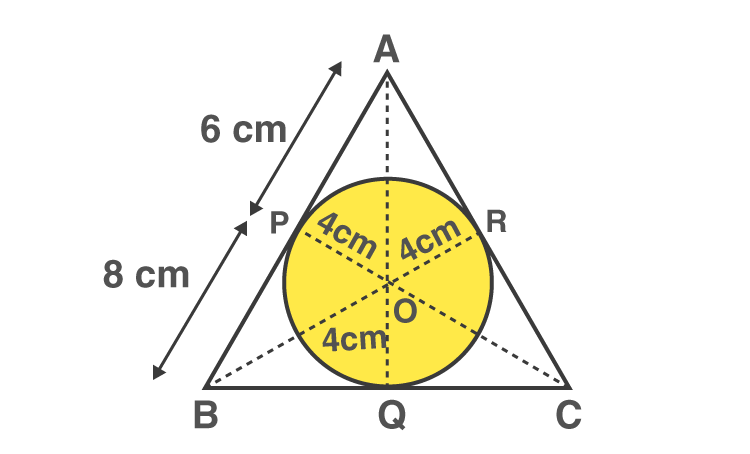# CBSE Class 10 Maths Board Exam: Important 2 marks questions

Central Board of Secondary Education (CBSE) has issued the official examination date for class 10th board exams. This exam is crucial from the examination aspect, as it helps us in obtaining the desired stream for forthcoming studies.

Students who wish to get into an engineering line requires to excel in the maths examination. The subject requires a proper understanding and skills to score well in final board exams. As two marks questions are direct types and can be framed from any small topic, so practicing the given questions can help acquire a good score. We at BYJU’S provide class 10th with Important 2 marks questions to practice and prepare for the maths board examination. If students want to practice more then, they can solve the CBSE 10th Sample Papers.

Students preparing for CBSE Class 10 Maths Board Examination are suggested to practice the given problems for Mathematics:

Important 2 Marks Questions for Class 10 Maths Board are as follows-

Q.1: Find the value of k for which the roots of the quadratic equation

$$\begin{array}{l}2x^{2}+ kx + 8 = 0\end{array}$$
, will have equal value.

Solution:

Given,

2x2 + kx + 8 = 0

Comparing with the standard form ax2 + bx + c = 0,

a = 2, b = k, c = 8

Condition for the equal roots is:

b2 – 4ac = 0

k2 – 4(2)(8) = 0

k2 – 64 = 0

k2 = 64

k = ±8

Q.2: Determine the AP whose third term is 5 and the seventh term is 9.

Solution:

Let a be the first term and d be the common difference of an AP.

Given,

Third term = 5

a + 2d = 5….(i)

Seventh term = 9

a + 6d = 9….(ii)

Subtracting (i) from (ii),

a + 6d – a – 2d = 9 – 5

4d = 4

d = 1

Substituting d = 1 in (i),

a + 2(1) = 5

a = 5 – 2 = 3

Therefore, the AP is: 3, 4, 5, 6,…

Q.3: Find a relation between x and y if the points A(x, y), B(-4, 6) and C(-2, 3) are collinear.

Solution:

Let the given points be:

A(x, y) = (x1, y1)

B(-4, 6) = (x2, y2)

C(-2, 3) = (x3, y3)

If three points are collinear then the area of the triangle formed by these points is 0.

i.e. (½) |x1(y2 – y3) + x2(y3 – y1) + x3(y1 – y2)| = 0

(½) |x(6 – 3) + (-4)(3 – y) + (-2)(y – 6)| = 0

x(3) – 4(3 – y) – 2(y – 6) = 0

3x – 12 + 4y – 2y + 12 = 0

3x + 2y = 0

Or

x = -2y/3

Q.4: In the figure, two tangents TP and TQ are drawn to a circle with centre O from an external point T, prove that ∠PTQ = 2OPQ.Solution:

Given that two tangents TP and TQ are drawn to a circle with centre O from an external point T

Let ∠PTQ = θ.

Now, by using the theorem “the lengths of tangents drawn from an external point to a circle are equal”, we can say TP = TQ. So, TPQ is an isosceles triangle.

Thus,

∠TPQ = ∠TQP = ½ (180°− θ ) = 90° – (½) θ

By using the theorem, “the tangent at any point of a circle is perpendicular to the radius through the point of contact”, we can say ∠OPT = 90°

Therefore,

∠OPQ = ∠OPT – ∠TPQ = 90° – [90° – (½) θ]

∠OPQ = (½)θ

∠OPQ = (½) ∠PTQ

⇒ ∠PTQ = 2 ∠OPQ.

Hence proved.

Q.5: A piece of wire 22 cm long is bent into the form of an arc of a circle subtending an angle of 60° at its centre. Find the radius of the circle. [Use π = 22/7]

Solution:

Length of the arc = Length of wire

(θ/360°) 2πr = 22

(60°/360°) × 2 × (22/7) × r = 22

(⅙) × (2/7) × r = 1

r = (7/2) × 6

r = 21

Therefore, the radius of the circle is 21 cm.

Q.6: If a number x is chosen at random from the numbers -3, -2, -1, 0, 1, 2, 3, what is the probability that x2 ≤ 4?

Solution:

Sample space = S = {-3, -2, -1, 0, 1, 2, 3}

n(S) = 7

Let E be the event of choosing a number x such that x2 ≤ 4.

i.e. x ≤ ±2

E = {-2, -1, 0, 1, 2}

n(E) = 5

P(E) = n(E)/n(S) = 5/7
Hence, the required probability is 5/7.

Q.7: The larger of two supplementary angles exceeds the smaller by 18°. Find the angles.

Solution:

Let x and (x + 18°) be the supplementary angles.

That means,

x + (x + 18°) = 180°

2x = 180° – 18°

2x = 162°

x = 162°/2

x = 81°

Now, x + 18° = 81° + 18° = 99°

Therefore, the supplementary angles are 81° and 99°.

Q.8: Find the mean of the following distribution:

 Class 3 – 5 5 – 7 7 – 9 9 – 11 11 – 13 Frequency 5 10 10 7 8

Solution:

 Class Frequency (fi) Midpoint (xi) fixi 3 – 5 5 4 20 5 – 7 10 6 60 7 – 9 10 8 80 9 – 11 7 10 70 11 – 13 8 12 96 Total ∑fi = 40 ∑xi = 40 ∑fixi = 326

Mean = ∑fixi/ ∑fi

= 326/40

= 8.15

Therefore, the mean of the given distribution is 8.15.

Q.9: Given that √2 is irrational, prove that (5 + 3√2) is an irrational number.

Solution:

Let (5 + 3√2 ) be a rational number.

5 + 3√2 = p/q (Where q ≠ 0 and p and q are co- prime numbers)

3√2 =  (p/q) – 5

3√2 =  (p – 5q )/q

√2 =  (p – 5q )/ 3q

p and q are integers and q ≠ 0

Thus, (p -5q) / 3q is rational number.

Also, √2 is a rational number.

However, it is given that √2  is an irrational number.

This is a contradiction, and thus, our assumption that (5 + 3√2 ) be a rational number is wrong.

That means (5+3√2) is an irrational number.

Hence proved.

Q.10: Find the value of p, for which one root of the quadratic equation px2 – 14x + 8 = 0 is 6 times the other.

Solution:

px2 – 14x + 8 = 0

Let α and 6α be the roots of the given quadratic equation.

Sum of the roots = -coefficient of x/coefficient of x2

α + 6α = -(-14)/p

7α = 14/p

α  = 2/p….(i)

Product of roots = constant term/coefficient of x2

(α)(6α) = 8/p

2 = 8/p

Substituting α = 2/p from (i),

6 × (2/p)2 = 8/p

24/p2 = 8/p

3/p = 1

p = 3

Therefore, the value of p is 3.

### Important 2 Marks Questions for Class 10 Maths for Practice

1. Two different dice are tossed together. Find the probability:(i) of getting a doublet(ii) of getting a sum of 10, of the numbers on the two dice.
2. Sumit is 3 times as old as his son. Five years later, he shall be two and a half times as old as his son. How old is Sumit at present?
3. Find the area of a triangle whose vertices are given as (1, -1), (-4, 6) and (-3, -5).
4. Find the mode of the following data:

 CI 0 – 20 20 – 40 40 – 60 60 – 80 80 – 100 100 – 120 120 – 14- f 6 8 10 12 6 5 3
5. The probability of selecting a blue marble at random from a jar that contains only blue, black and green marbles is ⅕. The probability of selecting a black marble at random from the same jar is ¼. If the jar contains 11 green marbles, find the total number of marbles in the jar.
6. Find the sum of the first 8 multiples of 3.
7. A circle touches all four sides of a quadrilateral ABCD. Prove that AB + CD = BC + DA.
8. A line intersects the y-axis at the points P and Q respectively. If (2,-5) is the midpoint of PQ, then find the coordinates of P and Q.
9. Which term of the A.P. 8, 14, 20, 26, …….. Will be 72 more than its 41st term?
10. In the figure below, the radius of incircle of
$$\begin{array}{l}\Delta ABC\end{array}$$
of area 84 cm2 is 4 cm and the lengths of the segment AP and BP into which side AB is divided by the point of contact are 6 cm and 8 cm. Find the lengths of the sides AC and BC.1. Find the value of k for which the roots of the quadratic equation 2x^2+kx+8=0, will have equal value.
Solution of this question

• If the roots of quadratic equation are equal, then the discriminant is zero.
Therefore,
D = 0
b^2 – 4ac = 0
Here, a=2, b=k and c=8
k^2 – 4*2*8 = 0
k^2 – 64 = 0
k^2 = 64
k = ±8

• The value of k.

\bf{\red{\underline{\bf{Explanation\::}}}}
Explanation:

We have 2x² + kx + 8 = 0

A/q

Discriminate (D) = 0

a = 2
b = k
c = 8
Formula use :

\boxed{\bf{b^{2}-4ac=0}}}

\begin{gathered}\longrightarrow\sf{b^{2} -4ac=0}\\\\\longrightarrow\sf{(k)^{2} -4\times 2\times 8=0}\\\\\longrightarrow\sf{(k)^{2} -64=0}\\\\\longrightarrow\sf{(k)^{2} =64}\\\\\longrightarrow\sf{k=\pm\sqrt{64} }\\\\\longrightarrow\sf{\green{k=\pm8}}\end{gathered}
⟶b
2
−4ac=0
⟶(k)
2
−4×2×8=0
⟶(k)
2
−64=0
⟶(k)
2
=64
⟶k=±
64

⟶k=±8

Thus;

The value of k is ±8 .

• for equal roots
D=0 , b^2-4ac=0
[k]^2-4*2*8=0
k^2-64=0
k^2= 64
k = 8